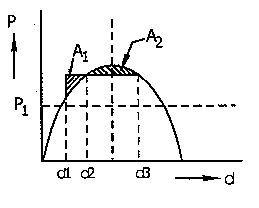# The inertia constant H of a synchronous condenser is

1.  greater than that of hydro generator

2.  greater than that of turbo alternator

3.  equal to that of the effective length of the line

4.  none of these

4

none of these

Explanation :
No Explanation available for this question

# Principle of Equal-area Criterion is to be applied to determine for a given initial load P1 the', maximum amount of sudden increase: in load p to maintain transient stability of a cylindrical rotor synchronous motor operating from an infinite bus. Applying this criterion (in each case the area A1= area A2 ). Then the correct diagram is

1.

2.

3.

4.

4Explanation :
No Explanation available for this question

# A power system has two synchronous generators The governor turbine characteristics or responding to the generators are P1 = 50(50 - f), P2=100(51-f) where f denotes the system frequency in Hz and P1 and P2 are respectively, the power outputs (in MW) of turbines 1 and 2. Assuming the generators and transmission network to be lossless, the system frequency for a total load of 400 MW is

1.  47.5 Hz

2.  48.0 Hz

3.  48.5 Hz

4.  49.0 Hz

4

48.0 Hz

Explanation :
No Explanation available for this question

# A power station consists of two sychronous generators A and B or ratings 250 MVA and 500 MVA with inertia 1.6 p.u. and 1 p.u. respectively on their own base MVA: ratings.The equivalent p.u.. constant for the system on 100 MVA common base is

1.  2.6

2.  0.615

3.  1.625

4.  9.0

4

0.615

Explanation :
No Explanation available for this question

# A 50 HZ, four-pole turbo alternator rated at 20 MVA, 13.2.kVhaa an inertia constant H = 4 kW see/kVA. The kinetic energy stored in the rotor at

1.  10 MJ

2.  80 MJ

3.  40 MJ

4.  20 MJ

4

80 MJ

Explanation :
No Explanation available for this question

# The inertia constants of two groups of machines which do not swing together are M1 and M2. The equivalent inertia constant of the system is

1.  M1+M2

2.  M1-M2 if M1>M2

3.  M1M2/M1+M2

4.  √M1M2

4

M1-M2 if M1>M2

Explanation :
No Explanation available for this question

# The inertia Constant Of a 100 MVA, 50 Hz, 4-pole generator is 10 MJ/MVA. If mechanical input to the machine is suddenly raised from 50 MW to 75 MW, then rotor acceleration will be

1.  225 electrical degree/s2

2.  22.5 electrical degree/s2

3.  125 electrical degree/s2

4.  12.5 electrical degree/s2

4

125 electrical degree/s2

Explanation :
No Explanation available for this question

# The steady-state stability limit of a synchronous generator can be increased by

1.  an increase in its reactance

2.  an increase in the excitation of the machine

3.  a decrease in the moment of inertia of the machine

4.  an increase in the moment of inertia of the machine

4

a decrease in the moment of inertia of the machine

Explanation :
No Explanation available for this question

# In the integral control of single area system, when system frequency error is reduced to zero

1.  integrator output and speed changer position attain a constant value

2.  integrator output increases but speed changer position also comes, down

3.  integrator output decreases and speed changer position also comes down

4.  integrator output decreases but speed changer position Moves up

4

integrator output decreases but speed changer position Moves up

Explanation :
No Explanation available for this question

1.  greater than transient stability limit

2.  equal to transient stability limit

3.  less than the transient stability limit

4.  none of these

4